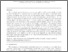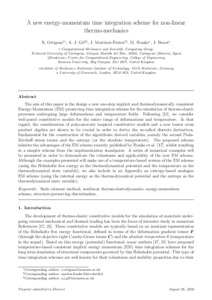# A new energy–momentum time integration scheme for non-linear thermo-mechanics

, Gil, A.J., Martínez-Frutos, J., Franke, M. and (2020) A new energy–momentum time integration scheme for non-linear thermo-mechanics. Computer Methods in Applied Mechanics and Engineering, 372:113395. ISSN 0045-7825 (doi:https://doi.org/10.1016/j.cma.2020.113395)Preview
PDF (Author's Accepted Manuscript)
29939 BONET_Energy-momentum_Time_Integration_Scheme_For_Non-linear_Thermo-mechanics_(AAM)_2020.pdf - Accepted Version

## Abstract

The aim of this paper is the design a new one-step implicit and thermodynamically consistent Energy–Momentum (EM)preserving time integration scheme for the simulation of thermo-elastic processes undergoing large deformations and temperature fields. Following Bonet et al. (2020), we consider well-posed constitutive models for the entire range of deformations and temperature. In that regard, the consideration of polyconvexity inspired constitutive models and a new tensor cross product algebra are shown to be crucial in order to derive the so-called discrete derivatives, fundamental for the construction of the algorithmic derived variables, namely the second Piola–Kirchoff stress tensor and the entropy (or the absolute temperature).The proposed scheme inherits the advantages of the EM scheme recently published by Franke et al. (2018), whilst resulting in a simpler scheme from the implementation standpoint. A series of numerical examples will be presented in order to demonstrate the robustness and applicability of the new EM scheme. Although the examples presented will make use of a temperature-based version of the EM scheme (using the Helmholtz free energy as the thermodynamical potential and the temperature as thethermodynamical state variable), we also include in an Appendix an entropy-based analogue EM scheme (using the internal energy as the thermodynamical potential and the entropy as the thermodynamical state variable).

Item Type: Article finite element method, nonlinear thermo-elastodynamics, energy–momentum scheme, structure-preserving discretisation T Technology > TA Engineering (General). Civil engineering (General) Vice-Chancellor's Group 28 Sep 2021 01:38 http://gala.gre.ac.uk/id/eprint/29939View Item